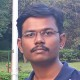How would you get all the points on a line?

I have detected the presence of lines in an image using the HoughLinesP() function. However, as an output, only the start and end points (coordinates) of the line are calculated by the function. I would however like to store all the points that fall on this line.

I initially thought of iterating from the starting coordinate to the end coordinate. But that gets quite complicated depending on the orientation of the line (horizontal, vertical, diagonal).

Any ideas on how I can achieve this?

edit retag close merge delete

Sort by » oldest newest most votedHi!

Opencv has Line Iterator function. Go through the documentation!

Here is a sample usage!

LineIterator it(img, pt1, pt2, 8);
for(int i = 0; i < it.count; i++, ++it)
{
Point pt= it.pos();
//Draw Some stuff using that Point pt
}
morei searched google "draw a line c++" and found this

the adaptation to your case is below

vector<Point> linePoints(int x0, int y0, int x1, int y1)
{
vector<Point> pointsOfLine;

int dx = abs(x1-x0), sx = x0<x1 ? 1 : -1;
int dy = abs(y1-y0), sy = y0<y1 ? 1 : -1;
int err = (dx>dy ? dx : -dy)/2, e2;

for(;;)
{
pointsOfLine.push_back(Point(x0,y0));
if (x0==x1 && y0==y1) break;
e2 = err;
if (e2 >-dx)
{
err -= dy;
x0 += sx;
}
if (e2 < dy)
{
err += dx;
y0 += sy;
}
}
return pointsOfLine;
}

to test it you can try the code below

//modified from https://github.com/Itseez/opencv/blob/master/apps/annotation/opencv_annotation.cpp
// move the mouse on the window and try left click and right click
#include <opencv2/core.hpp>
#include <opencv2/highgui.hpp>
#include <opencv2/imgproc.hpp>

using namespace std;
using namespace cv;

// Function prototypes
void on_mouse(int, int, int, int, void*);

vector<Point> linePoints(int x0, int y0, int x1, int y1)
{
vector<Point> pointsOfLine;

int dx = abs(x1-x0), sx = x0<x1 ? 1 : -1;
int dy = abs(y1-y0), sy = y0<y1 ? 1 : -1;
int err = (dx>dy ? dx : -dy)/2, e2;

for(;;)
{
pointsOfLine.push_back(Point(x0,y0));
if (x0==x1 && y0==y1) break;
e2 = err;
if (e2 >-dx)
{
err -= dy;
x0 += sx;
}
if (e2 < dy)
{
err += dx;
y0 += sy;
}
}
return pointsOfLine;
}

// Public parameters
Mat image(600, 800, CV_8UC3, Scalar(220, 220, 220));
vector<Point> points;
int roi_x0 = 0, roi_y0 = 0, roi_x1 = 0, roi_y1 = 0;
bool start_draw = false;

// Window name for visualisation purposes
const string window_name = "OpenCV Mouse Event Demo";

// FUNCTION : Mouse response for selecting objects in images
// If left button is clicked, start drawing a rectangle and circle as long as mouse moves
//If right button is clicked, look what will happen !
// Stop drawing once a new left click is detected by the on_mouse function
void on_mouse(int event, int x, int y, int, void *)
{
// Action when left button is clicked
if (event == EVENT_LBUTTONDOWN)
{

if (!start_draw)
{
roi_x0 = x;
roi_y0 = y;
start_draw = true;
}
else
{
roi_x1 = x;
roi_y1 = y;
start_draw = false;
vector<vector<Point> > contours;
contours.push_back(linePoints(roi_x0,roi_y0,roi_x1,roi_y1));
drawContours( image, contours, 0, Scalar(0, 0, 0), FILLED, 8 );
imshow(window_name, image);
}
}
// Action when mouse is moving
if ((event == EVENT_MOUSEMOVE) && start_draw)
{
// Redraw bounding box and rectangle
Mat current_view;
image.copyTo(current_view);

line(current_view, Point(roi_x0, roi_y0), Point(x, y), Scalar(0, 0, 255));
imshow(window_name, current_view);
}
}

int main(int argc, const char** argv)
{
// Init window interface and couple mouse actions
namedWindow(window_name, WINDOW_AUTOSIZE);
setMouseCallback(window_name, on_mouse);

imshow(window_name, image);

int key_pressed = 0;

do
{
// Keys for processing
// Based on the universal ASCII code of the keystroke: http://www.asciitable.com/
//      c = 99          add rectangle to current image
//      <ESC> = 27      exit program
key_pressed = 0xFF & waitKey(0);
if (key_pressed==99)
{
image=Scalar(220&rand(), 220, 220);
imshow(window_name, image);
}
}
// Continue as long as the <ESC> key has not been pressed
while (key_pressed != 27);

// Close down the window
destroyWindow(window_name);
return 0;
}
more